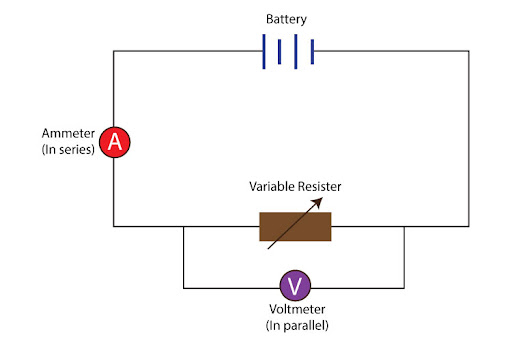# How do you use a Variable Resistor to determine values to show the relationship between I and V?

From the name itself, you can guess an important feature of the variable resistor: it’s resistance can vary. So with every new value of resistance, the amount of current flowing through will also change, causing the potential difference across it to also change. Meaning with different values of resistance, we will get a range of current and potential difference values.

So first thing’s first, we must construct a circuit:Two things to keep in mind:
• The ammeter must be placed in series with the variable resistor, so that it can record the exact value of current flowing (I) through it. Ideally, it should be placed after the positive terminal
• The voltmeter must be placed in parallel, across the variable resistor. That way, it can record the potential difference (V) across the two ends of the resistor.

Then, starting from a minimum value of resistance to a maximum value, record the corresponding values of current and potential difference. Make sure that each of the readings are steady before recording them; furthermore, make sure values of resistance are changed in equal increments to ensure consistency.

Then tabulate your data, and plot it on a graph. For an ohmic conductor, you should get a straight line passing through the origin, implying a direct proportionality relation between V and I. Any kind of curvature, and it’ll then be categorized as a non-ohmic conductor.